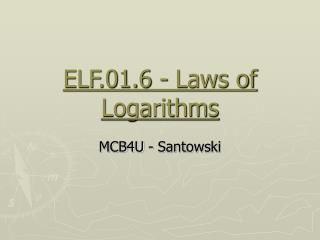DownloadDownload PresentationELF.01.6 - Laws of Logarithms

ELF.01.6 - Laws of Logarithms

Télécharger la présentationELF.01.6 - Laws of Logarithms

- - - - - - - - - - - - - - - - - - - - - - - - - - - E N D - - - - - - - - - - - - - - - - - - - - - - - - - - -
Presentation Transcript

1. ELF.01.6 - Laws of Logarithms MCB4U - Santowski

2. (A) Review • if f(x) = ax, find f -1(x) so y = ax then x = ay and now isolate y • in order to isolate the y term, the logarithmic notation or symbol or function was invented or created so we write x = ay as y = loga(x)

3. Consider this approach here in column 1 evaluate log5(625) = x 5x = 625 5x = 54 x = 4 Now try log5(625) = x tis way: log5(54) = x log5(5)4 = x Now I know from column 1 that the answer is 4 … so … log5(5)4 = 4 4 x log5(5) = 4 4 x 1 = 4 (B) Properties of Logarithms- Logarithms of Powers

4. (B) Properties of Logarithms- Logarithms of Powers • So if log5(625) = log5(5)4 = 4 x log5(5) • It would suggest a rule of logarithms  • logb(bx) = x • Which we can generalize  • logb(ax) = xlogb(a)

5. (C) Properties of Logarithms – Logs as Exponents • Consider the example • Recall that the expression log3(5) simply means “the exponent on 3 that gives 5”  let’s call that x • So we are then asking you to place that same exponent (the x) on the same base of 3 • Therefore taking the exponent that gave us 5 on the base of 3 (x ) onto a 3 again, must give us the same 5!!!! • We can demonstrate this algebraically as well

6. (C) Properties of Logarithms – Logs as Exponents • Let’s take our exponential equation and write it in logarithmic form • So becomes log3(x) = log3(5) • Since both sides of our equation have a log3 then x = 5 as we had tried to reason out in the previous slide • So we can generalize that

7. (D) Properties of Logarithms – Product Law • Express logam + logan as a single logarithm • We will let logam = x and logan = y • So logam + logan becomes x + y • But if logam = x, then ax = m and likewise ay = n • Now take the product (m)(n) = (ax)(ay) = ax+y • Rewrite mn=ax+y in log form  loga(mn)=x + y • But x + y = logam + logan • So thus loga(mn) = logam + logan

8. (E) Properties of Logarithms – Quotient Law • We could develop a similar “proof” to the quotient law as we did with the product law  except we ask you to express logam - logan as a single logarithm • As it turns out (or as is predictable), the simplification becomes as follows: • loga(m/n) = loga(m) – loga(n)

9. (F) Summary of Laws

10. (G) Examples • (i) log354 + log3(3/2) • (ii) log2144 - log29 • (iii) log30 + log(10/3) • (iv) which has a greater value • (a) log372 - log38 or (b) log500 + log2 • (v) express as a single value • (a) 3log2x + 2log2y - 4log2a • (b) log3(x+y) + log3(x-y) - (log3x + log3y) • (vi) log2(4/3) - log2(24) • (vii) (log25 + log225.6) - (log216 + log39)

11. (G) Internet Links • Logarithm Rules Lesson from Purple Math • College Algebra Tutorial on Logarithmic Properties from West Texas AM

12. (H) Homework • Nelson textbook, p125, Q1-14